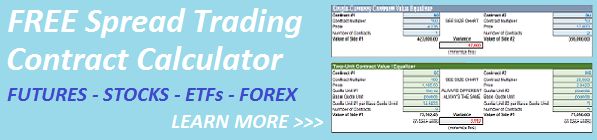Select Page< Back to Case 1

### How to Equalize Spread Legs When the Securities Have Different Quote Units

This is the base case where both securities are in the same currency and no currency transformation is required, but the two Quote Units are different.  You cannot equalize the two contracts without including a Quote Unit transformation to a Base Quote Unit.

Suppose you are spread trading two metals future contracts the CME’s COMEX, the Gold future (ticker GC) and the Copper future (ticker HG).  Both are denominated in USD but Gold is quoted in Troy Ounces and Copper is quoted in Pounds.  Let’s also say you want to normalize both contracts to Pounds (the Base Quote Unit).

For our example, let’s use these values:

• Currency = USD on both legs
• Currency Value vs. Base Currency (your trading currency) = not required
• Quote Unit = Troy Oz (GC) and Pounds (HG)
• Minimum Tick Value = 10 cents per Troy Ounce (GC) and 0.1 cents per Pound (HG)
• Contract Multiplier = 100 (GC) and 25,000 (HG)
• Price = 1185.50 (GC) and 2.842 (HG)
• Troy Ounces per Pound = 14.5833   To get accurate Quote Unit conversions GOOGLE “troy ounces per pound” or visit  http://www.metric-conversions.org

Here’s the contract calculation to normalize both legs.  Simply adjust the number of contracts on each leg to minimize the absolute variance in net exposures.

GC leg exposure = (100 x 1185.50 x contracts)/14.5833

HG leg exposure = 25,000 x 2.842 x contracts

Continue to Case 3:  How to Equalize Contracts Denominated in Different Currencies  >